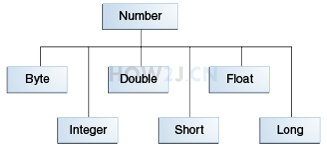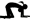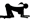5分3秒

封装类

package digit; public class TestNumber { public static void main(String[] args) { int i = 5; //把一个基本类型的变量,转换为Integer对象 Integer it = new Integer(i); //把一个Integer对象，转换为一个基本类型的int int i2 = it.intValue(); } }
```package digit;

public class TestNumber {

public static void main(String[] args) {
int i = 5;

//把一个基本类型的变量,转换为Integer对象
Integer it = new Integer(i);
//把一个Integer对象，转换为一个基本类型的int
int i2 = it.intValue();

}
}
```

Number类

Byte,Short,Integer,Long,Float,Doublepackage digit; public class TestNumber { public static void main(String[] args) { int i = 5; Integer it = new Integer(i); //Integer是Number的子类，所以打印true System.out.println(it instanceof Number); } }
```package digit;

public class TestNumber {

public static void main(String[] args) {
int i = 5;

Integer it = new Integer(i);
//Integer是Number的子类，所以打印true
System.out.println(it instanceof Number);
}
}
```

基本类型转封装类

package digit; public class TestNumber { public static void main(String[] args) { int i = 5; //基本类型转换成封装类型 Integer it = new Integer(i); } }
```package digit;

public class TestNumber {

public static void main(String[] args) {
int i = 5;

//基本类型转换成封装类型
Integer it = new Integer(i);

}
}
```

封装类转基本类型

package digit; public class TestNumber { public static void main(String[] args) { int i = 5; //基本类型转换成封装类型 Integer it = new Integer(i); //封装类型转换成基本类型 int i2 = it.intValue(); } }
```package digit;

public class TestNumber {

public static void main(String[] args) {
int i = 5;

//基本类型转换成封装类型
Integer it = new Integer(i);

//封装类型转换成基本类型
int i2 = it.intValue();

}
}
```

自动装箱

package digit; public class TestNumber { public static void main(String[] args) { int i = 5; //基本类型转换成封装类型 Integer it = new Integer(i); //自动转换就叫装箱 Integer it2 = i; } }
```package digit;

public class TestNumber {

public static void main(String[] args) {
int i = 5;

//基本类型转换成封装类型
Integer it = new Integer(i);

//自动转换就叫装箱
Integer it2 = i;

}
}
```

自动拆箱

package digit; public class TestNumber { public static void main(String[] args) { int i = 5; Integer it = new Integer(i); //封装类型转换成基本类型 int i2 = it.intValue(); //自动转换就叫拆箱 int i3 = it; } }
```package digit;

public class TestNumber {

public static void main(String[] args) {
int i = 5;

Integer it = new Integer(i);

//封装类型转换成基本类型
int i2 = it.intValue();

//自动转换就叫拆箱
int i3 = it;

}
}
```

int的最大值，最小值

int的最大值可以通过其对应的封装类Integer.MAX_VALUE获取
package digit; public class TestNumber { public static void main(String[] args) { //int的最大值 System.out.println(Integer.MAX_VALUE); //int的最小值 System.out.println(Integer.MIN_VALUE); } }
```package digit;

public class TestNumber {

public static void main(String[] args) {

//int的最大值
System.out.println(Integer.MAX_VALUE);
//int的最小值
System.out.println(Integer.MIN_VALUE);

}
}
```

练习-装箱拆箱Or姿势不对,事倍功半! 点击查看做练习的正确姿势
1. 对byte,short,float,double进行自动拆箱和自动装箱

2. byte和Integer之间能否进行自动拆箱和自动装箱

3. 通过Byte获取byte的最大值

答案-装箱拆箱2分11秒 本视频采用html5方式播放，如无法正常播放，请将浏览器升级至最新版本，推荐火狐，chrome，360浏览器 如果装有迅雷，播放视频呈现直接下载状态，请调整 迅雷系统设置-基本设置-启动-监视全部浏览器 (去掉这个选项)

package digit; public class TestNumber { public static void main(String[] args) { // 1. 对byte,short,float,double进行自动拆箱和自动装箱 byte b = 1; short s = 2; float f = 3.14f; double d = 6.18; // 自动装箱 Byte b1 = b; Short s1 = s; Float f1 = f; Double d1 = d; // 自动拆箱 b = b1; s = s1; f = f1; d = d1; // 2. byte和Integer之间能否进行自动拆箱和自动装箱 Integer i1 = b; //不能把byte直接自动装箱成Integer b = new Integer(1); //也不能把Integer自动拆箱成 byte // 3. 通过Byte获取byte的最大值 System.out.println(Byte.MAX_VALUE); } }

HOW2J公众号，关注后实时获知布最新的教程和优惠活动，谢谢。2019-05-29 关于byte和Integer之间是否可以自动装箱or拆箱以及我自己遇到的问题
 旺旺君 关于 JAVA 基础-数字与字符串-装箱拆箱 的提问 在eclipse试了一下 1.byte通过类型强转可以实现自动装箱，但是拆箱需要调用byteValue方法。 2.小数类型在进行装箱时也需要强转，但是拆箱时为什么可以直接赋值(没有使用Value方法)？ ```package digit;   public class TestNumber {       public static void main(String[] args) {           int a = 1;                   byte b = 2;                   short c = 3;                   float d = 4;                   double e = 5;           Byte i2 = b;         byte b2 = i2; //      byte通过类型强转实现自动装箱，但是拆箱需要调用byteValue方法         Integer i22 = (int)b;         byte b3 = i22.byteValue();                   Short i3 = c;         short c1 = i3;                   Float i4 = d;         float d1 = i4;                   Double i5 = e;         double e1 = i5;           //      小数在进行装箱时也需要强转，但是拆箱时却可以直接赋值(没有使用Value方法)         Integer i44 = (int)e;         double d2 = i44;                   Integer i55 = (int)e;         double e2 = i55;   //      通过Byte.MAX(MIN)_VALUE获取最值                       System.out.println(Byte.MAX_VALUE);   //      把一个基本变量转化为Integer对象         Integer i1 = new Integer(a);   //      把一个Integer对象转化为一个基本类型的变量         int a1 = i1.intValue();   //      Integer是Number的子类，故打印结果为true         System.out.println(i1 instanceof Number);   //      通过Integer。MAX(MIN)_VALUE获取最值         System.out.println(Integer.MIN_VALUE);     }   }```

1 个答案

wcx5683521 答案时间：2019-06-07

2019-05-29 关于byte和Integer之间是否可以自动装箱or拆箱以及我自己遇到的问题
 旺旺君 关于 JAVA 基础-数字与字符串-装箱拆箱 的提问 在eclipse试了一下 1.byte通过类型强转可以实现自动装箱，但是拆箱需要调用byteValue方法。 2.小数类型在进行装箱时也需要强转，但是拆箱时为什么可以直接赋值(没有使用Value方法)？ ```package digit; public class TestNumber { public static void main(String[] args) { int a = 1; byte b = 2; short c = 3; float d = 4; double e = 5; Byte i2 = b; byte b2 = i2; // byte通过类型强转实现自动装箱，但是拆箱需要调用byteValue方法 Integer i22 = (int)b; byte b3 = i22.byteValue(); Short i3 = c; short c1 = i3; Float i4 = d; float d1 = i4; Double i5 = e; double e1 = i5; // 小数在进行装箱时也需要强转，但是拆箱时却可以直接赋值(没有使用Value方法) Integer i44 = (int)e; double d2 = i44; Integer i55 = (int)e; double e2 = i55; // 通过Byte.MAX(MIN)_VALUE获取最值 System.out.println(Byte.MAX_VALUE); // 把一个基本变量转化为Integer对象 Integer i1 = new Integer(a); // 把一个Integer对象转化为一个基本类型的变量 int a1 = i1.intValue(); // Integer是Number的子类，故打印结果为true System.out.println(i1 instanceof Number); // 通过Integer。MAX(MIN)_VALUE获取最值 System.out.println(Integer.MIN_VALUE); } }```

2019-05-17 写作业
2019-05-13 ZZ交作业 装箱拆箱
2019-04-22 交作业
2019-04-22 加油周一
2019-04-17 旋转跳跃
2019-04-10 编译器的时候没有报错为什么运行的时候有这个错误？
2019-03-06 交作业交作业~
2019-01-13 作业参考
2019-01-04 交作业
2018-12-10 装箱拆箱！
2018-11-21 装箱和拆箱 主要应用在什么地方呢？？？
2018-11-12 交作业
2018-09-17 交作业交作业交作业交作业交作业交作业交作业
2018-08-30 按在地上摩擦~
2018-08-29 int 可以吧Byte自动拆箱，其他都不行
2018-08-27 我们一起成长~
2018-05-13 交作业
2018-05-07 交作业
2018-03-07 提交作业啊.
2018-03-05 自动拆箱这个怎么解释
2017-12-12 经过后面的学习，我胡汉三回来写个小记
2017-12-04 作业提交
2017-10-11 只有把Byte 自动拆箱到int可以。
2016-11-01 可以直接把Byte拆箱到int耶
2016-05-12 直接输出Integer类型不行吗，为什么要转换为int类型呢